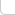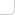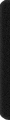# 某岛

… : "…アッカリ～ン . .. . " .. .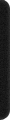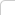January 30, 2013

# SRM 565

## DIV 1 Level 3. UnknownTree

### Brief description:

… 给定 3 + n 个结点和三组距离标号。。表示其他 n 个结点分别到这 3 个结点的距离。。
。。问所有满足这三组距离标号的树的方案总数。。

### Analysis:

（直接抄官方题解了。。这个题对我还是太难了。。a). 就算知道要逐步 reduce 到 1 个特殊结点的情况。。但是也不会做。。思维连贯性太大。。b). 有一些细节就算知道写错了也不知道怎么 cha …

http://apps.topcoder.com/wiki/display/tc/SRM+565

It is one problem with many complications. In cases like this, it may be a good idea to first try easier versions of the problem. For example, there are three special vertices: A, B, C. What if there was just one of them?

#### If there was only one special vertex

This easier problem is so easier, that it has in fact appeared as a topcoder problem before. In the division 1 medium slot. Let us take the special vertex A as the root of the tree. Then we just need to count, given a list of distances dist[i] of each vertex i to the root, the number of ways to set the edges.

https://www.shuizilong.com/house/archives/srm-474/

The solution is quite simple. Each vertex i must have only one parent (possibly the root). For each vertex i, the choices available for its parent are: a) The root. b) Any vertex j such that dist[j] < dist[i]. We can connect the vertex directly to the root, creating a single edge with weight dist[i] or we can connect it to another vertex that is dist[j] away to the root by creating an edge with weight (dist[i] - dist[j]). We cannot pick a vertex that has the same distance to root as i, because edges with weight 0 are not allowed. Note that these choices are independent from each other. This means that the result is equal to the product of (1 + number of vertices with dist[j] < dist[i]) for each i. As you can see, this version is approachable. We could try to find a way to reduce the real problem to this version. It is not going to be simple. Let us first try to see what can we do when there are two special vertices.

```    // The version with one root. d[] holds the d to the root.
Int f1(VI& d){
// Can be done in O(n), but O(n*n) is fast enough.
int n = SZ(d); Int res = 1; REP(i, n){
Int t = 1; REP(j, n) t += d[j] < d[i];
res *= t;
}
return res;
}
```

#### If there were two special vertices

Imagine a problem in which for each node, we know the distances to special vertices A and B. Let us use B as a root. The following observation is useful too: From the root (B), there may be many edges, but only one edge will be the edge that takes us closer to A. Let us call all the nodes reachable from this edge a branch; A’s branch.

We can reinterpret the problem as deciding whether to place each of the nodes in A’s branch or outside A’s branch. For the nodes outside A’s branch, we already know their distances to the root (B) the number of ways to count them is actually a instance of the subproblem that had only one special vertex. The nodes that belong to A’s branch, including A can be seen as a new instance of the two-special nodes problem: Just use the node that is closest to B as a new root.We cannot just try each possible combination of which nodes go to the branch and which do not. It would take too much time and there are many times in which the distances are such that it is not possible to take one of the decisions, or any. What can help simplify this problem is to assume that the minimum simple distance between A and B (AB) is known. This distance, combined with the known distances between each node to A and B actually determines whether or not the node belongs to the branch.Note that the dashed lines in the image do not necessarily represent single edges, but possibly multiple nodes connected by a path of edges that total each of
the given distances. Assume that the node i is outside A‘s branch. Then the distance from A to i must be equal to: AB + distanceB[i]. If this equality is not true, then it is impossible for node i to be outside A‘s branch. If the equality holds, it is impossible for node i to be
inside A‘s branch. All of the distances used in the equality are fixed so there is actually no choice to make.

There is an issue that might be easy to miss. It is that if the equality does not hold, there is a chance that the whole setting is not valid. If the equality does not hold, then we know that node i should belong to A‘s branch, but there might be reasons for it to be impossible to have this node in that branch. If that happens, then we have a contradiction and the whole case is invalid. The following is an invalid case:Imagine if the distances between i and B and between i and A were so low that it would not match what we know about AB. This leads us to a contradiction, node i cannot be simultaneously so close to A and B. We want the sum of these two distances to be at least equal AB. Note that this sum can be larger:Those both cases are valid, in each of them the sum of the distances is greater than AB.

That is the way in which fixing AB to a value can help us, as it alone decides which nodes belong outside and inside A‘s branch. Then we
can calculate the results for both sub-problems. Note that one of the new sub-problems is a new instance of the original problem: Given A and (a new) B, and the distances of each of the other nodes to those vertices, calculate the number of ways to assign the edges. This time, the new distance between the new A and B can be found from the previous ones. There will be a point in which the new B will be equal to A. This is the base case for the recursion. Then just verify that the distances match (else it is an invalid case). Then solve the 1 special vertex version.

```    Int f2(VII& d){

int n = SZ(d); if (n>1 && d.fi == d.fi) return 0;
FOR(i, 1, n) d[i].fi -= d.fi;

VI bO; if (!d.se){
FOR(i, 1, n){
if (d[i].fi != d[i].se) return 0;
bO.PB(d[i].fi);
}
return f1(bO);
}

VII bA; int ba = d.se; FOR(i, 1, n){
int ia = d[i].se, ib = d[i].fi;
if (ia - ba == ib) bO.PB(ib);
else {
if (ia + ib < ba) return 0;
bA.PB(d[i]);
}
}

return f1(bO) * f2(bA);
}
```

#### Three special vertices

When we have three special vertices, things get more complicated. Let us try to reuse the logic about branches. Then we need to think of
a single node i that divides the graph in such a way that A, B and C will each have its own branch. Basically the idea is as follows:Once again, the dashed lines could possibly represent whole paths of nodes. This assumption that there
is a node i that behaves as a center for the graph is not always true. There are special cases like the following:In those cases, one of the special nodes lies in the middle of the path between the other two. These special cases do not allow there
to be a single node i that behaves as the center. Let us treat the two variations of the problem separately.

#### Variation with a fixed center i

This time, we assume that one of the non-special vertices i acts like a center. We can just try each of the O(n) such vertices as a potential center and sum all the ways to make the tree using that center. Note that for each tree, at most one of the vertices can do this. This vertex is the unique intersection between the paths AB, AC and BC.

Then we can just use the same logic as the 2-special vertices case. We can use i as the root. There is a branch for each of A, B or C and some vertices lying outside the three branches. There is a decision to make: A vertex can go to one of the branches or outside. The outside vertices then make a instance of the version of the problem with 1 special node. More importantly: each of the branches and all its nodes makes a version of the problem with 2 special nodes. For example, C‘s branch can be reduced to the 2 nodes problem with A=C and B=i.

What is left is to decide where each vertex should go. This time we already know the distances between i and A, i and B and i and C. This will yield four cases.

If we were to assume a node j lies outside all of the branches, the following happens:(distanceA[j] – distanceA[i]) must be equal to (distanceB[j] – distanceB[i]) and (distanceC[j] – distanceC[i]). These values will be equal to the distance between j and i. If these subtractions are all equal (and greater than 0) then node j must lie outside all of the branches and its distance to i is known (useful when solving the 1-special node case).

The other case is when node i belongs to one of the three branches. Let us use C‘s branch as an example. The other cases are similar by symmetry.This time, (distanceA[j] – distanceA[i]) must be equal to (distanceB[j] – distanceB[i]). (But different to (distanceC[j] – distanceC[i]), else it would be the other case). This would mean that the node must belong to C’s branch. The distance between i and j is (distanceA[j] – distanceA[i]) and the distance between i and C is distanceC[i]. Which allows us to solve the 2 special nodes case. Note that the sum of the distances between j and i and between j and C must be at least distanceC[i], similar to the condition in the 2 special nodes case (Its solution can do this check for us).

If instead (distanceA[j] – distanceA[i]) and (distanceC[j] – distanceC[i]) were equal, then node j must lie inside B’s branch. If (distanceB[j] – distanceB[i]) and (distanceC[j] – distanceC[i]) are equal then j must be inside A’s branch. What happens if all of the subtractions are different? This would mean the case is invalid as the node j cannot lie outside of the branches and inside any of them.

This approach should count all the trees in which one of the nodes that are not special acts as a center.

```    Int f31(VI& dA, VI& dB, VI& dC){

Int res; int n = SZ(dA); REP(o, n){

VII bA, bB, bC; VI bO;

bA.PB(MP(0, dA[o])), bA.PB(MP(dA[o], 0));
bB.PB(MP(0, dB[o])), bB.PB(MP(dB[o], 0));
bC.PB(MP(0, dC[o])), bC.PB(MP(dC[o], 0));

bool valid = true; REP(i, n) if (i != o){
int da = dA[i] - dA[o], db = dB[i] - dB[o], dc = dC[i] - dC[o];
if (da > 0 && da == db && db == dc) bO.PB(da);
else if (db > 0 && db == dc) bA.PB(MP(db, dA[i]));
else if (dc > 0 && dc == da) bB.PB(MP(dc, dB[i]));
else if (da > 0 && da == db) bC.PB(MP(da, dC[i]));
else {
valid = false;
break;
}
}

if (valid){
SRT(bA), SRT(bB), SRT(bC);
res += f1(bO) * f2(bA) * f2(bB) * f2(bC);
}
}

return res;
}
```

#### C is between A and B

The cases in which one of the special nodes lies between the other two are different, but can all be solved with the same logic. Let us assume C is the vertex that is between A and B. The main complication is that the distances between A and C and B and C are unknown. For now let us assume they are known. Let us use C as root. This once again allows us to divide nodes by branches. A node i can belong to A‘s branch, B‘s branch and outside. Then we can again reduce to the 2-nodes case or the 1-node case.

This time, if distanceC[i] is equal to (distanceA[i] – AC) and (distanceA[i] – AB), then the vertex lies outside both branches. If distanceC[i] is only equal to (distanceA[i] – AC), then the node lies in B‘s branch. If distanceC[i] is only equal to (distanceB[i] – AB), then i lies inside A‘s branch.This time, if distanceC[i] is equal to (distanceA[i] – AC) and (distanceA[i] – AB), then the vertex lies outside both branches. If distanceC[i] is only equal to (distanceA[i] – AC), then the node lies in B‘s branch. If distanceC[i] is only equal to (distanceB[i] – AB), then i lies inside A‘s branch.

This only works because we assumed to know AC and BC. Unfortunately, we cannot just try all possible values from 1 to 1012 for them. The final observation is to notice that there are only few candidates for valid pairs (AC, BC), and they depend on the already-known distances from each node and A, B and C.

Remember how the distances AC and BC determine a node’s placement? (In which branch). It can work the other
way. For each node i, try the possibilities: Place it outside both branches, in A‘s branch or in B‘s branch. Each of these settings will require a specific
pair (AC, BC) to work.

For example, if we assume node i is outside both branches, then distanceC[i] must be equal to (distanceA[i] – AC) and (distanceA[i] – AB), thus we can just get AC and AB from those equations.

There are O(n) candidates for pairs (AC, BC) we can just try them all and sum the results for each. Two trees with
different distances (AC, BC) will always be different.

```    Int f32(VI& dL, VI& dR, VI& dM){

set<PII> L; int n = SZ(dM); REP(i, n){
L.insert(MP(abs(dL[i]-dM[i]), abs(dR[i]-dM[i])));
if (dL[i]-dM[i]>0) L.insert(MP(dL[i]-dM[i], dR[i]+dM[i]));
if (dR[i]-dM[i]>0) L.insert(MP(dL[i]+dM[i], dR[i]-dM[i]));
}

Int res; ECH(it, L){

int lm = it->fi, mr = it->se;
VII bA, bB; VI bO;

bA.PB(MP(0, lm)), bA.PB(MP(lm, 0));
bB.PB(MP(0, mr)), bB.PB(MP(mr, 0));

bool valid = true; REP(i, n){
int dl = dL[i] - lm, dr = dR[i] - mr, dm = dM[i];
if (dm == dl && dm == dr) bO.PB(dm);
else if (dl == dm) bB.PB(MP(dm, dR[i]));
else if (dr == dm) bA.PB(MP(dm, dL[i]));
else {
valid = false;
break;
}
}

if (valid) {
SRT(bA), SRT(bB);
res += f1(bO) * f2(bA) * f2(bB);
}
}

return res;
}
```

http://paste.ubuntu.com/5572292/